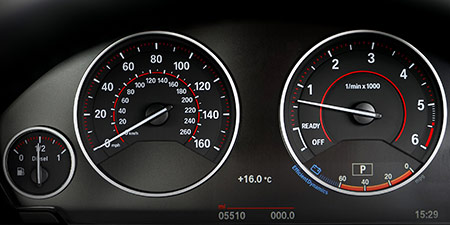# Kilometers per hour (km/h) to Yards per hour (yd/h)

Thema Units of speed ﹣ Converter Kilometers per hour to Yards per hour

Here you can convert the Speed unit Kilometers per hour into the unit Yards per hour and vice versa you can convert Yards per hour into Kilometers per hour. By clicking the "Swap units" icon, you will always obtain the desired conversion in the calculation result, i.e., km/h to yd/h or yd/h to km/h. With the following calculator you can also calculate any other Speed unit.

## Info about "Kilometers per hour"

Kilometers per hour is used as a standard unit in transportation, therefore it is used in traffic or to measure the travel speed of vehicles. For example, a commercial aircraft flies at a speed of 1,000 km/h then it covers a distance of 1,000 kilometers per hour.

The unit is defined as follows: An object moving at 1 "km/h" per hour will cover one kilometer. "km/h" is approved to use in the international system of units (SI).

## Info about "Yards per hour"

The length unit "Yard" is commonly used in the Anglo-American countries. For example, speeds are represented by yard per unit of time.

The unit is defined as follows: An object moving at 1 "Yard per hour" per hour will cover a distance of one yard. Therefore, 1 yard is equivalent to 0.9144 meters (3 feet).

## Basis for conversion Kilometers per hour (km/h) to Yards per hour (yd/h) and vice versa

The abbreviation for the "Speed unit Kilometers per hour" is km/h. The abbreviation for the "Speed unit Yards per hour" is yd/h.

## Formula for the conversion of Kilometers per hour (km/h) to Yards per hour (yd/h) and vice versa

The calculation from Kilometers per hour to Yards per hour shall be made using the following conversion formula:

Conversion formula Kilometers per hour to Yards per hour

Determine the number of Yards per hour from Kilometers per hour

Kilometers per hour × 1093.6132983377

## Formula for the conversion of Yards per hour (yd/h) to Kilometers per hour (km/h)

The calculation from Yards per hour to Kilometers per hour shall be made using the following conversion formula:

Conversion formula Yards per hour to Kilometers per hour

Determine the number of Kilometers per hour from Yards per hour

Yards per hour × 0.0009144

## Overview table : How many Kilometers per hour are in Yards per hour ?

Kilometers per hour km/h ⇒ Yards per hour yd/h
0.01 km/h  are  10.93613 yd/h
0.02 km/h  are  21.87226 yd/h
0.03 km/h  are  32.80839 yd/h
0.04 km/h  are  43.74453 yd/h
0.05 km/h  are  54.68066 yd/h
0.06 km/h  are  65.61679 yd/h
0.07 km/h  are  76.55293 yd/h
0.08 km/h  are  87.48906 yd/h
0.09 km/h  are  98.42519 yd/h
0.10 km/h  are  109.36133 yd/h
0.20 km/h  are  218.72266 yd/h
0.30 km/h  are  328.08399 yd/h
0.40 km/h  are  437.44531 yd/h
0.50 km/h  are  546.80664 yd/h
0.60 km/h  are  656.16797 yd/h
0.70 km/h  are  765.52930 yd/h
0.80 km/h  are  874.89063 yd/h
0.90 km/h  are  984.25196 yd/h
1 km/h  corresponds to  1 093.61329 yd/h
2 km/h  are  2 187.22659 yd/h
3 km/h  are  3 280.83989 yd/h
4 km/h  are  4 374.45319 yd/h
5 km/h  are  5 468.06649 yd/h
6 km/h  are  6 561.67979 yd/h
7 km/h  are  7 655.29308 yd/h
8 km/h  are  8 748.90638 yd/h
9 km/h  are  9 842.51968 yd/h
10 km/h  are  10 936.13298 yd/h
20 km/h  are  21 872.26596 yd/h
30 km/h  are  32 808.39895 yd/h
40 km/h  are  43 744.53193 yd/h
50 km/h  are  54 680.66491 yd/h
60 km/h  are  65 616.79790 yd/h
70 km/h  are  76 552.93088 yd/h
80 km/h  are  87 489.06386 yd/h
90 km/h  are  98 425.19685 yd/h
100 km/h  are  109 361.32983 yd/h
200 km/h  are  218 722.65966 yd/h
300 km/h  are  328 083.98950 yd/h
400 km/h  are  437 445.31933 yd/h
500 km/h  are  546 806.64916 yd/h
600 km/h  are  656 167.97900 yd/h
700 km/h  are  765 529.30883 yd/h
800 km/h  are  874 890.63867 yd/h
900 km/h  are  984 251.96850 yd/h
1 000 km/h  are  1 093 613.29833 yd/h

## Overview table : How many Yards per hour are in Kilometers per hour ?

Yards per hour yd/h ⇒ Kilometers per hour km/h
0.02 yd/h  are  0.00001 km/h
0.03 yd/h  are  0.00002 km/h
0.04 yd/h  are  0.00003 km/h
0.05 yd/h  are  0.00004 km/h
0.06 yd/h  are  0.00005 km/h
0.07 yd/h  are  0.00006 km/h
0.08 yd/h  are  0.00007 km/h
0.09 yd/h  are  0.00008 km/h
0.10 yd/h  are  0.00009 km/h
0.20 yd/h  are  0.00018 km/h
0.30 yd/h  are  0.00027 km/h
0.40 yd/h  are  0.00036 km/h
0.50 yd/h  are  0.00045 km/h
0.60 yd/h  are  0.00054 km/h
0.70 yd/h  are  0.00064 km/h
0.80 yd/h  are  0.00073 km/h
0.90 yd/h  are  0.00082 km/h
1 yd/h  corresponds to  0.00091 km/h
2 yd/h  are  0.00182 km/h
3 yd/h  are  0.00274 km/h
4 yd/h  are  0.00365 km/h
5 yd/h  are  0.00457 km/h
6 yd/h  are  0.00548 km/h
7 yd/h  are  0.00640 km/h
8 yd/h  are  0.00731 km/h
9 yd/h  are  0.00823 km/h
10 yd/h  are  0.00914 km/h
20 yd/h  are  0.01828 km/h
30 yd/h  are  0.02743 km/h
40 yd/h  are  0.03657 km/h
50 yd/h  are  0.04572 km/h
60 yd/h  are  0.05486 km/h
70 yd/h  are  0.06400 km/h
80 yd/h  are  0.07315 km/h
90 yd/h  are  0.08229 km/h
100 yd/h  are  0.09144 km/h
200 yd/h  are  0.18288 km/h
300 yd/h  are  0.27432 km/h
400 yd/h  are  0.36576 km/h
500 yd/h  are  0.45720 km/h
600 yd/h  are  0.54864 km/h
700 yd/h  are  0.64008 km/h
800 yd/h  are  0.73152 km/h
900 yd/h  are  0.82296 km/h
1 000 yd/h  are  0.91440 km/h

## Source information

As source for the information in the "Units of speed" category, we have used in particular:

## Last update on May 3, 2022

The pages of the "Units of speed" category were last editorially reviewed by Stefan Banse on May 3, 2022. They all correspond to the current status.

### Previous changes on October 9, 2021

• November 9, 2020: Publication of the Speed converter
• Editorial revision of all texts in this category